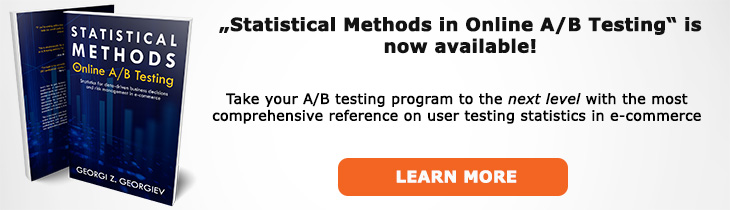# What does "Confidence Interval" mean?

Definition of Confidence Interval in the context of A/B testing (online controlled experiments).

## What is a Confidence Interval?

Aliases: CI

A confidence interval is such a random interval that when constructed over repeated tests of the same type with different data it will cover the true value of the parameter of interest a set proportion/percentage of the time. The desired proportion is called a confidence level and is usually expressed as percentages, e.g. 90%, 95%, 99%. Constructing CIs is an important part of estimation procedures.

A confidence interval can also be viewed from the point of hypothesis testing due to the duality between confidence intervals and p-values. Any null hypothesis defined over a set of values not covered by the confidence interval can be rejected at least at significance level equal to 1 minus the confidence level, e.g. if a 95% confidence interval covers the values from 0.01 to +∞ then a null that covers the values from -∞ to 0.005 can be rejected at the 1-0.95 = 0.05 level or higher.

It should be noted that confidence intervals lose their interpretation once a particular CI is realized from the data. It would be incorrect to say that the true value lies within a specific confidence interval (say from 0.01 to 0.04) with probability XX%: the value is either covered by the interval or it is not covered. Like other frequentist measures the confidence level of confidence intervals refers to the statistical test itself and not to any particular hypothesis. A severity interpretation of CIs can allow them to maintain their relevance post-hoc.

It should also be noted that values within the interval should be treated equally: one cannot argue that one is more likely than another. Not doing so can lead to the interpretation that any particular value inside the interval is well-supported: in fact each individual value has very little support on its own.

A common pitfall when making claims relative to one of the bounds (confidence limits) of a two-sided 90% interval is to say something like "the values below the lower limit can be rejected at the 0.1 (1 - CI%) significance level". In fact, the values above the lower bound of a 90% confidence interval can be rejected at the 0.05 (1 - (1 - CI%)/2) significance level when using a one-tailed test/one-sided hypothesis. When you want to make claims about values laying below or above a certain point with a given level of confidence you should build the corresponding one-sided interval (with one of their limits being plus or minus infinity).

One-Tailed Test

Two-Tailed Test

Confidence Level

Confidence Limit

## Articles on Confidence Interval

Like this glossary entry? For an in-depth and comprehensive reading on A/B testing stats, check out the book "Statistical Methods in Online A/B Testing" by the author of this glossary, Georgi Georgiev.## Glossary Index by Letter

Select a letter to see all A/B testing terms starting with that letter or visit the Glossary homepage to see all.